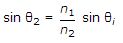# Online Civil Engineering Test - Elements of Remote Sensing TestLoading Test...

Instruction:

• This is a FREE online test. DO NOT pay money to anyone to attend this test.
• Total number of questions : 20.
• Time alloted : 30 minutes.
• Each question carry 1 mark, no negative marks.
• DO NOT refresh the page.
• All the best :-).

1.

The arrangement of terrain features which provides attributes: the shape, size and texture of objects, is called :

A.
 spectral variationB.
 spatial variationC.
 temporal variationD.
 None of these .2.

Due to pertubation of the orbit, satellite orbit parameters are frequently updated on measurements carried out by its

A.
 six ground stationsB.
 five ground stationsC.
 four ground stationsD.
 three ground stations3.

Which one of the following errors is produced by platform characteristics of the sensor ?

A.
 altitude variationB.
 altitudeC.
 orbit driftD.
 All of these4.

The spectral region of the electromagnetic radiation which passes through the atmosphere without much attenuation is known as:

A.
 ozone holeB.
 atmospheric windowC.
 ozone windowD.
 black hole5.

The remote sensing techniques applied for the earth's surface features, is generally confined to the following wave lengths :

A.
 0.4 to 1.3, 1.5 to 1.8, 2.2 to 2.6 μmB.
 2.2 to 2.6, 3.0 to 3.6, 4.2 to 5.0 μmC.
 4.2 to 5.0, 7.0 to 15.0 μm and 1 cm to 30 cmD.
 All of these6.

A perfectly black body :

A.
 is a diffuse emitterB.
 absorbs all the radiations of every wave lengthsC.
 emits power of every wave lengthD.
 All the above7.

Which one of the following parameters is accurate for DGPS.

A.
 Positional accuracies ~ 1 - 2 m if rover is less than 1-2 km from the reference stationB.
 Positional accuracies ~ 2 - 5m if rover is less than 2-5 fm from the reference stationC.
 Positional accuracies ~ 5 - 10 m if rover is less than 5-10 km from reference stationD.
 Positional accuracies ~ 5 - 10 m if rover is less than 25 km8.

The various stages occuring in GPS system are described below :
1. Generation of an output to the user
2. Detection of the GPS signal
3. Processing the data in the built-in-computer
4. Decoding the GPS signal.
The correct sequence of the stages is :

A.
 1, 2, 3, 4B.
 2, 3, 4, 1C.
 2, 4, 3, 1D.
 3, 1, 2 , 49.

The interaction of the electromagnetic radiation produced with a specific wave length to illuminate a target on the terrain for studying its scattered radiance, is called:

A.
 passive remote sensingB.
 active remote sensingC.
 neutral remote sensingD.
 None of these10.

The code based GPS receivers are generally used for:

A.
 Vehicle trackingB.
 Land navigationC.
 Trans movementD.
 All of these11.

The GPS space segment consists of Navigation Satellite Timing and Ranging whose number is :

A.
 8B.
 12C.
 16D.
 2412.

Which one of the following geometric errors of satellite sensors is random ?

A.
 scan skewB.
 panoramic distortionC.
 earth rotationD.
 altitude variation13.

The reflectance from a surface is called specular reflection if it follows: .

A.
 Snells's lawB.
 Lambert's cosine lawC.
 Planktan's lawD.
 All of these14.

According to the Snell's law if an electromagnetic wave is incident in a medium (refractive index n1) on an other medium (refractive index m2);

A.
 the angle of incidence is equal to the angle of refractionB.
 the angle of refraction (θr) is given byC.
 Both (a) and (b)D.
 neither (a) nor (b)15.

While propagating through homogeneous, isotropic media,

A.
 directions of both the fields are orthogonalB.
 both the fields are at right angles to the direction of propagation.C.
 both (a) and (b)D.
 niether (a) nor (b)16.

Which one of the following statements is correct ?

A.
 Each satellite of the GPS system transmits two L band signals.B.
 The two L band signals are modulated by the Pseudorandom binary codes (PN).C.
 The GPS receiver reads the specific code of the satellite.D.
 The PN code determines the time taken by the receiver to reach the receiver which is converted into distanceE.
 All of these17.

In sun-light water poor in phyto plank tons appears :

A.
 deep blueB.
 redC.
 greenD.
 yellow18.

The maximum absorption of solar radiation due to ozone occurs at wave lengths:

A.
 shorter than 0.3 μmB.
 shorter than 0.2 μmC.
 greater than 0.3 μmD.
 None of these19.

Pick up the correct statement from the following:

A.
 Water molecules and salt-ions in the ocean scatter the lower wave length moreB.
 An increase in the back scattering coefficient with shorter wave length gives a deep blue colour for clear oceanic waterC.
 The ratio of the upwelling irradiance just below the surface and downwelling irradiance just above the surface is called reflectance ratio.D.
 All of these20.

Snow albedo is affected by :

A.
 wave lengthB.
 grain sizeC.
 solar zenith angleD.
 snow pack thicknessE.
 All of these